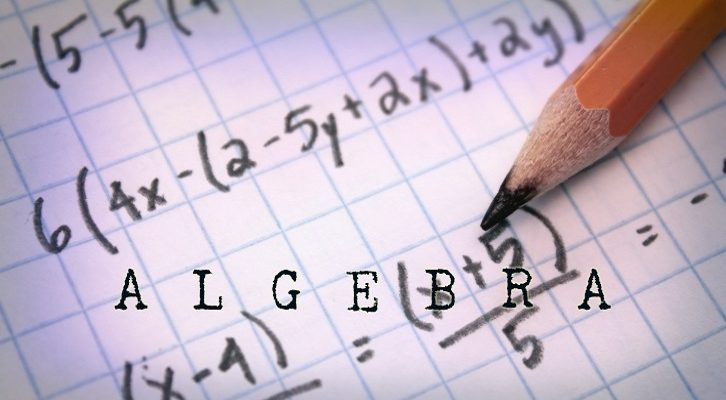# CAT Inequalities – Few questions

Have given three interesting questions from Inequalities.

Questions

1.  Find the range of x for which (x + 2) (x + 5) > 40.

2.  How many integer values of x satisfy the equation x( x + 2) (x + 4) (x + 6) < 200

3.  Find the range of x where ||x – 3| – 4| > 3.

1.  x < -10 or x > 3

2.  9 different values

3.  (-infinity, -4) or (2,4) or ( 10, infinity)

1.  Find the range of x for which (x + 2) (x + 5) > 40.

There are two ways of trying this one. We can expand and simplify this algebraically. x^2 + 7x + 10 > 40 or

x^2 + 7x – 30 > 0
(x + 10) (x -3) > 0
The roots are -10 and +3.
=> x should lie outside the roots.

Now, what is this based on?
There is a simple thumb rule for solving quadratic inequality
For any quadratic inequality ax2+ bx + c < 0
Factorize it as a(x – p) ( x – q) < 0

Whenever a is greater than 0, the above inequality will hold good if x lies between p and q.
a(x – p) (x – q) will be greater than 0, whenever x does not lie between p and q. In other words x should lie in the range ( -infinity, p) or (q, infinity)

Now, coming back to the question (x+10) (x -3) > 0
Or,x < -10 or x > 3

Second method: 5 * 8 = 40, -8 * -5 = 40
So, if x + 2 > 5 this will hold good => x > 3
If x + 2 is less than -8 also, this will hold good => x < -10. The first method is far more robust.

2.  How many integer values of x satisfy the equation x( x + 2) (x + 4) (x + 6) < 200

To begin with – 0, -2, -4 and -6 work. These are the values for which the left-hand side goes to zero.

There are 4 terms in the product. If all 4 are positive or all 4 are negative the product will be positive
The product can be negative only if exactly 1 or exactly 3 are negative. When 1 or 3 terms are negative, the product is clearly less than 200.

When x = -1, one term is negative
When x = -5, three terms are negative

So, adding these two numbers also to the set of solutions {-6, -5, -4, -2, -1, 0} satisfy the inequality.

Beyond this it is just trial and error.

Let us try x = 1. Product is 1 * 3 *  5 * 7 = 105. This works
x = -7 gives the same product. So, that also works.

So, the solution set is now refined to  {-7, -6, -5, -4, -2, -1, 0, 1}

x = 2 => Product is 2 * 4 * 6 * 8 = 8 * 48. Not possible. Any x greater than 1 does not work
x = -8 is also not possible. Any value of x less than -7 does not work.

So, the solution set stays as {-7, -6, -5, -4, -2, -1, 0, 1}

The one missing value in this sequence is -3. When x = -3, product becomes -3 * -1 * 1 * 3. = 9. This also holds good.
So, values {-7, -6,-5, -4, -3, -2, -1, 0, 1} hold good. 9 different values satisfy this inequality

3.  Find the range of x where ||x – 3| – 4| > 3

If we have an inequality |y| > 3, this will be satisfied if => y > 3 or y < -3.

So, the above inequality simplifies to two inequalities

Inequality I: | x – 3| – 4 > 3

| x – 3| – 4 > 3 => | x – 3 | > 7
x – 3 > 7 or x – 3 < -7
Or, x > 10 or x < -4

Of, x lies outside of  -4 and 10. Or, x can lie in the range ( – infinity, -4) or ( 10, infinity)

Inequality II: |x -3| – 4 < -3

|x – 3 | – 4 < – 3
=> | x – 3 | < 1
=> -1 < x – 3 < 1 or x lies between 2 and 4

So, final solution is given by the range
( – infinity, -4) or (2,4) or ( 10, infinity)

————————————————————————-

2iim – CAT Classes, Correspondence material.
CAT weekend batches @ Chennai
Weekend batches @ Anna Nagar from 24th November, @ Velachery from 25th November. @ Mylapore from 2nd December.